abstractmath.org 2.0
help with abstract math

Produced by Charles Wells     Revised 2016-11-04
Introduction to this website
website TOC    website index   blog


Category theory turns math inside-out. Definitions depend on nothing inside, but on everything outside. -- John Cook

This is a draft of the first part of an article on category theory.

During the last year or so, I have been monitoring the category theory questions on Math Stack Exchange. Some of the queries are clearly from people who do not have enough of a mathematical background to understand basic abstract reasoning, for example the importance of definitions and the difficulties described in the abmath artice on Dysfunctional attitudes and behaviors. Category theory has become important in several fields outside mathematics, for example computer science and database theory.

This article is intended to get people started in category theory by giving a very detailed definition of "category" and some examples described in detail with an emphasis on how the example fits the definition of category. That's all the present version does, but I intend to add some examples of constructions and properties such as the dual category, product, and other concepts that some of the inquirers on Math Stack Exchange had great difficulty with.

There is no way in which this article is a proper introduction to category theory. It is intended only to give beginners some help over the initial steps of understanding the subject, particularly the aspects of understanding that cause many hopeful math majors to fall off the Abstraction Cliff.

To be written.

# Definition of category

A category is a type of Mathematical structure consisting of two types of data, whose relationships are entirely determined by some axioms. After the definition is complete, I introduce several example categories with a detailed discussion of each one, explaining how they fit the definition of category.

## Axiom 1: Data

A category consists of two types of data: objects and arrows.

### Notes for Axiom 1

• You will see in the section on Examples of categories that every definition of a category $\mathsf{C}$ starts by specifying what the objects of $\mathsf{C}$ are and what the arrows of $\mathsf{C}$ are. That is what Axiom I requires.
• An object of a category can be any kind of mathematical object. It does not have to be a set and it does not have to have elements.
• Arrows of a category are also called morphisms. You may be familiar with "homomorphisms", "homeomorphisms" or "isomorphisms", all of which are functions. This does not mean that a "morphism" in an arbitrary category is a function.

## Axiom 2: Domain and codomain

Each arrow of a category has a domain and a codomain, each of which is an object of the category.

### Notes for Axiom 2

• The domain and the codomain of an arrow may or may not be the same object.
• Each arrow has only one domain and only one codomain.
• If $f$ is an arrow with domain $A$ and codomain $B$, that fact is typically shown either by the notation "$f:A\to B$" or by a diagram like this:

•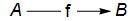• Warning: The notation "$f:A\to B$" is like that used for functions. This notation may be used in any category, but it does not imply that $f$ is a function or that $A$ and $B$ have elements.
• For an arrow $f:A\to B$, the notation "$\text{dom}(f)$" refers to $A$ and "$\text{cod}(f)$" refers to $B$.
• For a given category $\mathsf{C}$, the collection of all the arrows with domain $A$ and codomain $B$ may be denoted by
• "$\text{Hom}(A,B)$" or
• "$\text{Hom}_\mathsf{C}(A,B)$" or
• "$\mathsf{C}(A,B)$".
• Some newer books and articles in category theory use the name source for domain and target for codomain. This usage has the advantage that a newcomer to category theory will be less likely to think of an arrow as a function.

## Axiom 3: Composition

If $f$ and $g$ are arrows in a category for which $\text{cod}(f)=\text{dom}(g)$, as in this diagram:then there is a unique arrow with domain $A$ and codomain $C$ called the composite of $f$ and $g$.

### Notes for Axiom 3

• The unique arrow required by Axiom 3 may be denoted by "$g\circ f$" or "$gf$". "$g\circ f$" is more explicit, but "$gf$" is much more commonly used by category theorists.
• Many constructions in categories may be shown by diagrams, like the one used just above.
• The diagramis said to commute if $h=g\circ f$.
• It is useful to think of $f$ followed by $g$ as a path in the diagram. Then a metaphor for composition is: Every path of length 2 has exactly one composite.
• It is customary in some texts in category theory to indicate that a diagram commutes by putting a gyre in the middle:• Note that the composite of the path that I described as "$f$ followed by $g$" is written as "$g\circ f$" or "$gf$", which seems backward. Nevertheless, the most common notation in category theory for the composite of "$f$ followed by $g$" is $gf$. Some authors in computer science write "$f;g$" for "$gf$" to get around this problem.
• The concept of category is an abstraction of the idea of function, and the composition of arrows is an abstraction of the composition of functions. It uses the same notation, "$g\circ f$". If $f$ and $g$ are set functions, then for an element $x$ in the domain of $f$, $(g\circ f)(x)=g(f(x))$
• But in arbitrary category, it may make no sense to evaluate an arrow $f$ at some element $x$; indeed, the domain of $f$ may not have elements at all, and then the statement "$(g\circ f)(x)=g(f(x))$" is meaningless.

## Axiom 4: Identity arrows

1. For each object $A$ of a category, there is an arrow denoted by $\mathsf{id}_A$.
2. $\textsf{dom}(\textsf{id}_A)=A$ and $\textsf{cod}(\textsf{id}_A)=A$.
3. For any object $B$ and any arrow $f:B\to A$, the diagram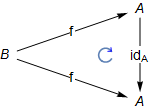commutes.
4. For any object $C$ and any arrow $g:A\to C$, the diagram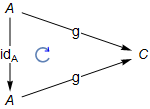commutes.

### Notes for Axiom 4

• The fact stated in Axiom 4(b) could be shown diagrammatically either asor as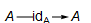• Facts (c) and (d) can be written in algebraic notation: For any arrow $f$ going to $A$,$\textsf{id}_A\circ f=f$and for any arrow $g$ coming from $A$,$g\circ \textsf{id}_A=g$
• There may be many arrows with domain and codomain both equal to $A$ (for example in the category $\mathsf{Set}$), but only one of them is $\textsf{id}_A$. It can be proved that $\textsf{id}_A$ is the unique arrow satisfying both (c) and (d) of the axiom.

## Axiom 5: Associativity

1. If $f$, $g$ and $h$ are arrows in a category for which $\text{cod}(f)=\text{dom}(g)$ and $\text{cod}(g)=\text{dom}(h)$, as in this diagram:then there is a unique arrow $k$ with domain $A$ and codomain $D$ called the composite of $f$, $g$ and $h$.

2. In the diagram below, the two triangles containing $k$ must both commute.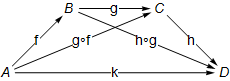### Notes for Axiom 5

• Axiom 5b requires that $h\circ(g\circ f)=(h\circ g)\circ f$(which both equal $k$), which is the usual algebraic notation for associativity.
• Note that the top two triangles commute by Axiom 3.
• The associativity axiom means that we can get rid of parentheses and write $k=h\circ f\circ g$just as we do for addition and multiplication of numbers.
• In my opinion the notation using categorical diagrams communicates information much more clearly than algebraic notation does. In particular, you don't have to remember the domains and codomains of the functions -- they appear in the picture. I admit that diagrams take up much more space, but now that we read math stuff on a computer screen instead of on paper, space is free.

# Examples of categories

For these examples, I give a detailed explanation about how they fit the definition of category.

## Example 1: MyFin

This first example is a small, finite category which I have named $\mathsf{MyFin}$ ("my finite category"). It is not at all an important category, but it has advantages as a first example.

• It's small enough that you can see all the objects and arrows on the screen at once, but big enough not to be trivial.
• The objects and arrows have no properties other than being objects and arrows. (Some of the other examples involve familiar math objects.)
• So in order to check that $\mathsf{MyFin}$ really obeys the axioms for a category, you can use only the skeletal information given here. As a result, you must really understand the axioms!

A correct proof will be based on axioms and theorems.
The proof can be suggested by your intuitions,
but intuitions are not enough.
When working with $\mathsf{MyFin}$ you won't have any intuitions!

### A diagram for $\mathsf{MyFin}$

This diagram gives a partial description of $\mathsf{MyFin}$.Now let's see how to make the diagram above into a category.

### Axiom 1: Data

• The objects of $\mathsf{MyFin}$ are $A$, $B$, $C$ and $D$.
• The arrows are $f$, $g$, $h$, $j$, $k$, $r$, $s$, $u$, $v$, $w$ and $x$.
• You can regard the letters just listed as names of the objects and arrows. The point is that at this stage all you know about the objects and arrows are their names.
• If you prefer, you can think of the arrows as the actual arrows shown in the $\mathsf{MyFin}$ diagram.
• Our definition of $\mathsf{MyFin}$ is an abstract definition. You may have seen multiplication tables of groups given in terms of undefined letters. (If you haven't, don't worry.) Those are also abstract definitions.
• Our other definitions of categories involve math objects you actually know something about.

### Axiom 2: Domain and Codomain

• The domains and codomains of the arrows are shown by the diagram above.
• For example, $\text{dom}(r)=A$ and $\text{cod}(r)=C$, and $\text{dom}(v)=\text{cod}(v)=B$.

### Axiom 3: Composition

Showing the $\mathsf{MyFin}$ diagram does not completely define $\mathsf{MyFin}$. We must say what the composites of all the paths of length 2 are.

• In fact, most of them are forced, but two of them are not.
• We must have $g\circ f=r$ because $r$ is the only arrow possible for the composite, and Axiom 3 requires that every path of length 2 must have a composite.
• For the same reason, $h\circ g=s$.
• All the paths involving $u$, $v$, $w$ and $x$ are forced:
• (p1) $u\circ u=u$, $v\circ v=v$, $w\circ w=w$ and $x\circ x=x$.
• (p2) $f\circ u=f$, $r\circ u=r$, $j\circ u=j$ and $k\circ u=k$. You can see that, for example, $f\circ u=f$ by opening up the loop on $f$ like this: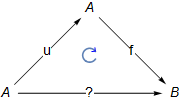There is only one arrow going from $A$ to $B$, namely$f$, so $f$ has to be the composite $f\circ u$.
• (p3) $v\circ f=f$, $g\circ v=g$ and $s\circ v=s$.
• (p4) $w\circ g=g$, $w\circ r=r$ and $h\circ w=h$.
• (p5) $x\circ h=h$, $x\circ s=s$, $x\circ j=j$ and $x\circ k=k$.
• For $s\circ f$ and $h\circ r$, we have to choose between $j$ and $k$ as composites. Since $s\circ f=(h\circ g)\circ f$ and $h\circ r=h\circ (g\circ f)$, Axiom 3 requires that we must chose one of $j$ and $k$ to be both composites.

Definition: $s\circ f=h\circ r=j$.

If we had defined $s\circ f=h\circ r=k$ we would have a different category, although one that is "isomorphic" to $\mathsf{MyFin}$ (you have to define "isomorphic" or look it up.)

### Axiom 5: Associativity

• Since we have already required both $(h\circ g)\circ f$ and $h\circ(g\circ f)$ to be $k$, composition is associative.

## Example 2: IntegerDiv

• This example uses familiar mathematical objects -- positive integers.
• The arrows are not functions that can be applied to elements, since integers do not have elements.

### Axiom 1: Data

• The objects of IntegerDiv are all the positive integers.
• Suppose $m$ and $n$ are positive integers:
• If $m$ divides $n$, there is exactly one arrow from $m$ to $n$. I will call this arrow $\textsf{mdn}$. (This is my notation. There is no standard notation for this category.) For example there is one arrow from $2$ to $6$, denoted by $\textsf{2d6}$.
• If $m$ does not divide $n$, there is no arrow from $m$ to $n$.

### Axiom 2: Domain and codomain

The arrow denoted by $\textsf{mdn}$ has domain $m$ and codomain $n$.

##### Example##### Example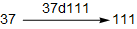##### Examplewhich may also be shown as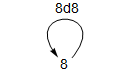### Axiom 3: Composition

The composite of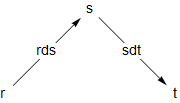must be $\textsf{rdt}$, since that is the only arrow with domain $r$ and codomain $t$.

This fact can also be written this way: $\mathsf{sdt}\circ\textsf{rds}=\textsf{rdt}$

### Axiom 4: Identity arrows

The composites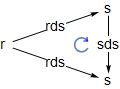andmust commute since the arrows shown are the only possible arrows with the domains and codomains shown. In other words, $\textsf{id}_\textsf{r}=\textsf{rdr}$ and $\textsf{id}_\textsf{s}=\textsf{sds}$.

### Axiom 5: Associativity

In the diagram below,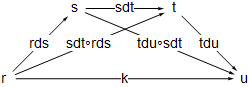there is only one arrow from one integer to another, so $\textsf{k}$ must be both $\textsf{tdu}\circ(\textsf{sdt}\circ\textsf{rds})$ and $(\textsf{tdu}\circ\textsf{sdt})\circ\textsf{rds}$ as required.

## Example 3: The category of Sets

In this section, I define the category $\mathsf{Set}$ (that is standard terminology in category theory.) This example will be very different from $\mathsf{MyFin}$, because it involves known mathematical objects -- sets and functions.

### Axiom 1: Data

• Every set is an object of $\mathsf{Set}$ and nothing else is.
• Every function between sets is an arrow of $\mathsf{Set}$ and nothing else is an arrow of $\mathsf{Set}$.

### Axiom 2: Domain and codomain

For a given function $f$, $\text{dom}(f)$ is the domain of the function $f$ in the usual sense, and $\text{cod}(f)$ is the codomain of $f$ in the usual sense. (See Functions: specification and definition for more about domain and codomain.)

#### Examples

• Let $f:\mathbb{R}\to\mathbb{R}$ be the function defined by $f(x):=x^2$. Then the arrow $f$ in $\mathsf{Set}$ satisfies $\text{dom}(f)= \mathbb{R}$ and also $\text{cod}(f)=\mathbb{R}$.
• Let $j:\{1,2,3\}\to\{1,2,3,4\}$ be defined by $j(1):=1$, $j(2):=4$ and $j(3):=3$. Then $\text{dom}(j)=\{1,2,3\}$ and $\text{cod}(j)=\{1,2,3,4\}$.

### Axiom 3: Composition

The composite of $f:A\to B$ and $g:B\to C$ is the function $g\circ f:A\to C$ defined by $\text{(DC)}\,\,\,\,\,\,\,\,\,\,(g\circ f)(a):=g(f(a))$

#### Note

Many other categories have a similar definition of composition, including categories whose objects are math structures with underlying sets and whose arrows are structure-preserving functions between the underlying sets. But be warned: There are many useful categories whose arrows do not evaluate at an element of an object because the objects don't have elements. In that case, (DC) is meaningless. This is true of $\mathsf{MyFin}$ and $\mathsf{IntegerDiv}$.

### Axiom 4: Identity arrows

For a set $A$, the identity arrow $\textsf{id}_A:A\to A$ is, as you might expect, the identity function defined by $\textsf{id}_A(a)=a$ for every $a\in A$. We must prove that these diagrams commute:The calculations below show that they commute. They use the definition of composite given by (DC).

• For any $b\in B$, $(\textsf{id}_A\circ f)(b)=\textsf{id}_A(f(b))=f(b)$
• For any $a\in A$, $(g\circ \textsf{id}_A)(a)=g(\textsf{id}_A(a))=g(a)$

Note: In $\mathsf{Set}$, there are generally many arrows from a particular set $S$ to itself (for example there are $4$ from $\{1,2\}$ to itself), but only one is the identity arrow.

### Axiom 5: Associativity

Composition of arrows in $\mathsf{Set}$ is associative because function composition is associative. Suppose we have functions as in this diagram:We must show that the two triangles containing $k$ in this diagram commute:In algebraic notation, this requires showing that for every element $a\in A$,$(h\circ(g\circ f))(a))=((h\circ g)\circ f)(a)$

The calculation below does that. It makes repeated use of Definition (DC) of composition. For any $a\in A$,$\begin{equation} \begin{split} \big(h\circ (g\circ f)\big)(a) & = h\big((g\circ f)(a)\big) \\ & = h\big(g(f(a))\big) \\ & = (h\circ g)(f(a)) \\ & = \big((h\circ g)\circ f\big)(a) \end{split} \end{equation}$

## Example 4: The category of Monoids

• This definition makes repeated use of the fact that a homomorphism of monoids is a set function. Specifically, if $(S,\Delta)$ and $(T,\nabla)$ are monoids with identities $e_S$ and $e_{T}$, a homomorphism $h:S\to T$ must be a set function that satisfies the following two axioms: $\text{(ME)}\,\,\,\,\,\,\,\,h(e_S)=e_T$ and for all elements $s, s'$ of $S$, $\text{(MM)}\,\,\,\,\,\,\,\,h(s\Delta s')=h(s)\nabla h(s')$
• The category of monoids may be denoted by $\mathsf{Mon}$.

### Axiom 1: Data

• Every monoid is an object of the category of monoids, and nothing else is.
• If $f$ is a homomorphism of monoids, then $f$ is an arrow of the category of monoids, and nothing else is.

### Axiom 2: Domain and codomain

If $(S,\Delta)$ and $(T,\nabla)$ are monoids and $f:(S,\Delta)\to(T,\nabla)$ is a homomorphism of monoids, then the domain of $f$ is $(S,\Delta)$ and the codomain of $f$ is $(T,\nabla)$.

#### Notes

• Since $f$ takes elements of the set $S$ to elements of the set $T$, it is also an arrow in the category $\mathsf{Set}$. In general, very few functions from $S$ to $T$ will be monoid homomorphisms from $(S,\Delta)$ to $(T,\nabla)$.
• Many authors do not distinguish between $f$ regarded as an arrow of $\mathsf{Mon}$ and $f$ regarded as an arrow of $\mathsf{Set}$. Others may write $U(f)$ for the arrow in $\mathsf{Set}$. "$U$" stands for "underlying functor", also called "forgetful functor".

### Axiom 3: Composition

The composite ofis the composite $g\circ f$ as set functions: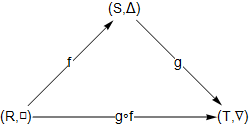It is necessary to check that $g\circ f$ is a monoid homomorphism. The following calculation shows that it preserves the monoid operation; it makes repeated use of equations (DC) and (MM).

The calculation: For elements $r$ and $r'$ of $R$,\begin{align*} (g\circ f)(r\,{\square}\, r') &=g\left(f(r\, {\square}\, r')\right)\,\,\,\,\,\text{(DC)}\\ &=g\left(f(r) {\scriptstyle\, \Delta}\, f(r')\right)\,\,\,\,\,\text{(MM)}\\ &=g(f(r)){\,\nabla}\, g(f(r'))\,\,\,\,\text{(MM)}\\ &=(g\circ f)(r){\,\nabla}\,(g\circ f)(r')\,\,\,\,\,\text{(DC)} \end{align*}

The fact that $g\circ f$ preserves the identity of the monoid is shown in the next section.

### Axiom 4: Identity arrows

For a monoid $(S,\Delta)$, the identity function $\text{id}_S:S\to S$ preserves the monoid operation $\Delta$, because $\text{id}_S(s\Delta s')=s\Delta s'$ by definition of the identity function, and that is $\text{id}_S(s)\Delta \text{id}_S(s')$ for the same reason.

The required diagrams below must commute because the set functions commute and, by Axiom 3, the set composition of a monoid homomorphism is a monoid homomorphism.We also need to show that $g\circ f$ as inpreserves identities. This calculation proves that it does; it uses (DC) and (ME)

\begin{align*} (g\circ f)(\text{id}_R) &=g(f((\text{id}_R))\\ &=g(\text{id}_S)\\ &=\text{id}_T \end{align*}

### Axiom 5: Associativity

The diagramin the category $\mathsf{Set}$ commutes, so the diagrammust also commute.

# References

All these references are available on line.